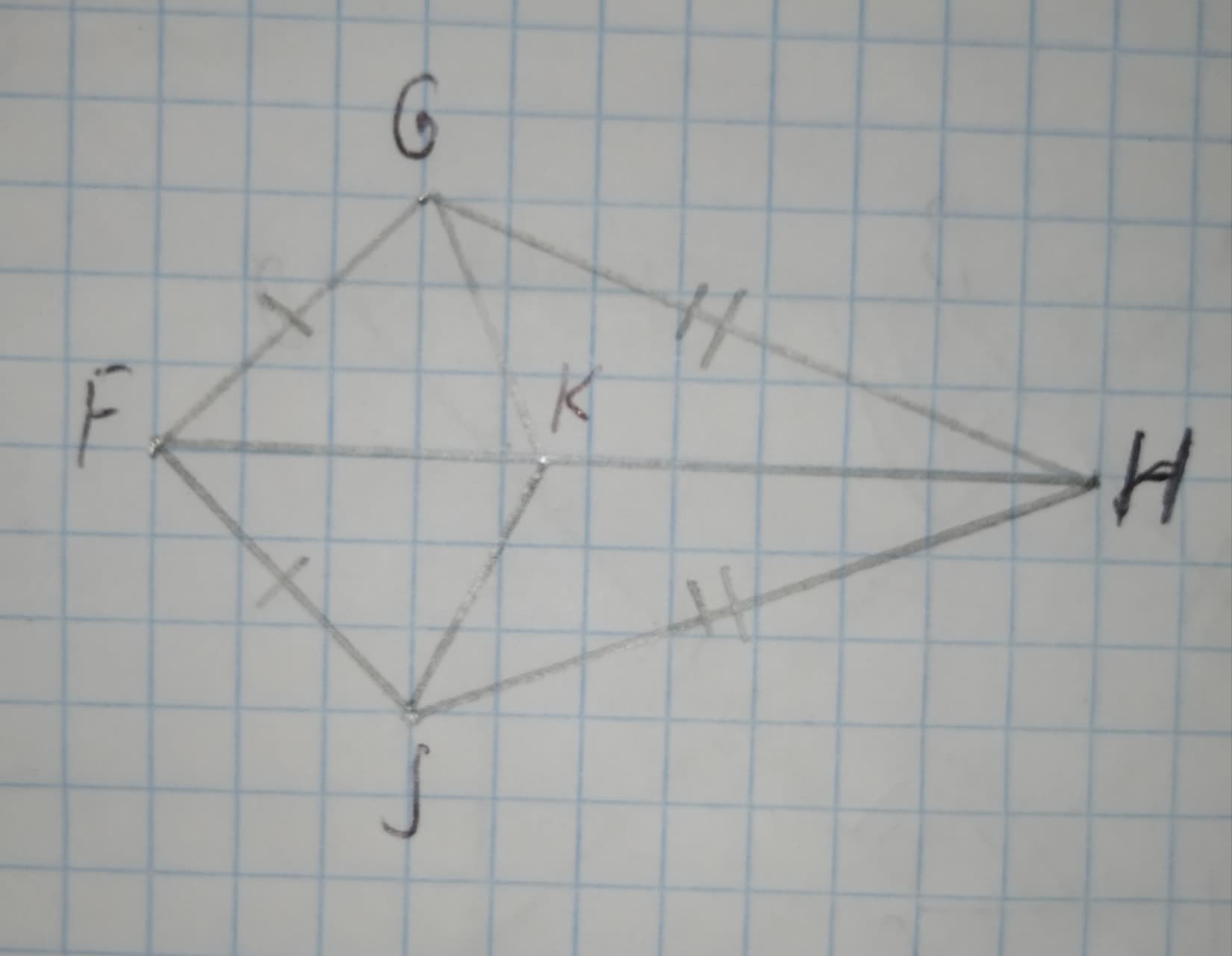# Select all the true statements. 12210203832.jpg A./_\FGK ~= /_\FJK B./_GKH ~= JKH C.bar(FG) ~= bar(KG) D./_GFH ~= /_JFH E./_\GKH ~= /_\JKH F.bar(FG) ~= bar(JK)rocedwrp 2020-10-23 Answered
Select all the true statements.A.$\mathrm{△}FGK\stackrel{\sim }{=}\mathrm{△}FJK$
B.$\mathrm{\angle }GKH\stackrel{\sim }{=}JKH$
C.$\stackrel{―}{FG}\stackrel{\sim }{=}\stackrel{―}{KG}$
D.$\mathrm{\angle }GFH\stackrel{\sim }{=}\mathrm{\angle }JFH$
E.$\mathrm{△}GKH\stackrel{\sim }{=}\mathrm{△}JKH$
F.$\stackrel{―}{FG}\stackrel{\sim }{=}\stackrel{―}{JK}$
You can still ask an expert for help

## Want to know more about Congruence?

• Questions are typically answered in as fast as 30 minutes

Solve your problem for the price of one coffee

• Math expert for every subject
• Pay only if we can solve ithajavaF
Step 1
Given:Step 2
We have,
$In\mathrm{△}FGH\phantom{\rule{1em}{0ex}}\text{and}\phantom{\rule{1em}{0ex}}\mathrm{△}FJH$,
FG=FJ(Given)
GH=JH(Given)
FH=FH(Common side)
So, by SSS congruence criteria
$\mathrm{△}FGH\stackrel{\sim }{=}\mathrm{△}FJH$...(i)
A.$In\mathrm{△}FGK\phantom{\rule{1em}{0ex}}\text{and}\phantom{\rule{1em}{0ex}}\mathrm{△}FJK$,
FG=FJ(Given)
$\mathrm{\angle }GFK=\mathrm{\angle }JFK$ [Using(i)]
FK=FK(Common side)
So, by SAS congruence criteria
$\mathrm{△}FGK\stackrel{\sim }{=}\mathrm{△}FJK$
B.Similarity, using(i) and A, we get
$In\mathrm{△}GKH\phantom{\rule{1em}{0ex}}\text{and}\phantom{\rule{1em}{0ex}}\mathrm{△}JKH$,
GK=JK[Using(A)]
GH=JH(Given)
KH=KH(Common side)
So, by SSS congruence criteria
$\mathrm{△}GKH\stackrel{\sim }{=}\mathrm{△}JKH$
Hence, $\mathrm{\angle }GKH\stackrel{\sim }{=}\mathrm{\angle }JKH$
D.Using(i),$\mathrm{\angle }GFH\stackrel{\sim }{=}\mathrm{\angle }JFH$
E.Already proved in B, $\mathrm{△}GKH\stackrel{\sim }{=}\mathrm{△}JKH$
C & F. These two points are False.
Step 3
Hence the true statements are A, B, D, and E.